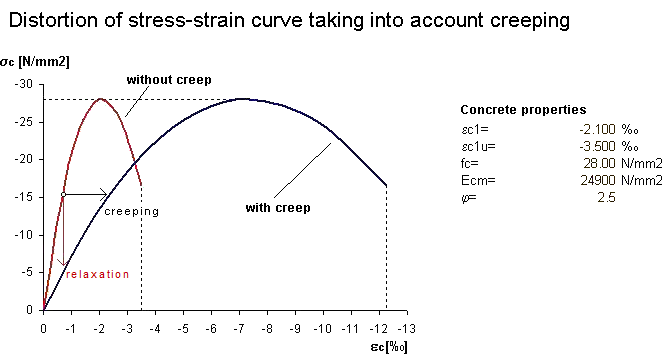# RF-CONCRETE Members – Online Manual Version 5

Online manuals, introductory examples, tutorials, and other documentation.

# 2.4.6 Creep and Shrinkage

### Creep and Shrinkage

Determining the coefficients for creep φ (t,t0) and shrinkage εc,s (t,ts) according to EN 1992-1-1, Annex B is described in Chapter 2.2.6.

Creep and shrinkage in the model are considered by calculation as described below.

Creeping

If the strains are known at the point of time t = 0 as well as any later point of time t, the factor for creeping φt can be specified as follows.

The equation is converted to the strain at the point of time t. This results in the following correlation, which is valid for uniform stresses (less than approx. 0.4 fck).

For stresses higher than approx. 0.4 fck, the strains increase disproportionately, resulting in the loss of the linearly assumed reference.

The calculation in RF-CONCRETE Members uses a common solution that is reasonable for construction purposes: The concrete's stress-strain curve is distorted by the factor (1+ φ).Figure 2.28 Distortion of stress-strain relation to determine creep effect

When taking account of creeping, uniform creep-producing stresses are assumed during the period of load application as shown in the figure above. Due to the neglect of stress redistributions, the deformation is slightly overestimated by this approach. In addition, this model comprises stress reduction only in parts as the change in strains (relaxation) is not taken into account: If we assume a linear elastic behavior, it would be possible to presume a proportionality and the horizontal distortion would also reflect the relaxation at a ratio of (1+ φ). This context, however, is lost for the nonlinear stress-strain relation.

This procedure thus represents an approximation. A reduction of stresses due to relaxation as well as nonlinear creep cannot or can only approximately be represented.

The creep coefficient φt applied in RF-CONCRETE Members is to be considered an effective creep coefficient. For calculations in the ultimate limit state, this means that the ratio of creep-producing and acting load must be taken into account. Therefore, the creep coefficients determined according to Chapter 2.2.6 must be adjusted as shown in the following equation.

Shrinkage

The question arises how distortions of the structural component relevant for the calculation are caused. The reason for this is the concrete's restrained reduction due to reinforcement. If the boundary conditions of common "slender" components with uniformly distributed shrinkage strain are assumed, component curvatures will occur only for asymmetric reinforcement distribution.

Therefore, the shrinkage can be represented by a pre-strain of concrete or steel. In detail, this means that the steel's "free strain" is restrained by a positive pre-strain of concrete. In the same way, it would be possible to model the component with a negative pre-strain of steel so that the concrete restrains the free strain of the pre-strained steel. Both variants show identical stress distributions while taking into account the respective pre-strain, but they differ significantly on the strain level: If steel is pre-strained, it is immediately evident from the strain's condition where zones of tension and compression due to shrinkage occur. If concrete is pre-strained, it is possible to make statements from the strain's condition concerning the concrete's actual reduction.

As the determination of deformations is most important for the calculation, it is of no interest whether the modeling in the stiffness determination is carried out by a positive pre-strain of concrete or a negative pre-strain of reinforcement.

RF-CONCRETE Members takes shrinkage strain by negative pre-strain of the reinforcing steel into account.